Important Questions: Direct and Inverse Proportions

# Important Questions: Direct and Inverse Proportions Notes | Study Mathematics (Maths) Class 8 - Class 8

## Document Description: Important Questions: Direct and Inverse Proportions for Class 8 2022 is part of Direct and Inverse Proportions for Mathematics (Maths) Class 8 preparation. The notes and questions for Important Questions: Direct and Inverse Proportions have been prepared according to the Class 8 exam syllabus. Information about Important Questions: Direct and Inverse Proportions covers topics like and Important Questions: Direct and Inverse Proportions Example, for Class 8 2022 Exam. Find important definitions, questions, notes, meanings, examples, exercises and tests below for Important Questions: Direct and Inverse Proportions.

Introduction of Important Questions: Direct and Inverse Proportions in English is available as part of our Mathematics (Maths) Class 8 for Class 8 & Important Questions: Direct and Inverse Proportions in Hindi for Mathematics (Maths) Class 8 course. Download more important topics related with Direct and Inverse Proportions, notes, lectures and mock test series for Class 8 Exam by signing up for free. Class 8: Important Questions: Direct and Inverse Proportions Notes | Study Mathematics (Maths) Class 8 - Class 8
 1 Crore+ students have signed up on EduRev. Have you?

Question 1: A mixture of paint is prepared by mixing 1 part of green pigments with 6 parts of the base. In the following table, find the parts of base needed to be added.

 Parts of green pigment 1 4 5 6 Parts of base 6 x1 x2 x3

Solution: Here, as the base increased, the required number of green pigments will also increase.
∴ The quantity vary directly: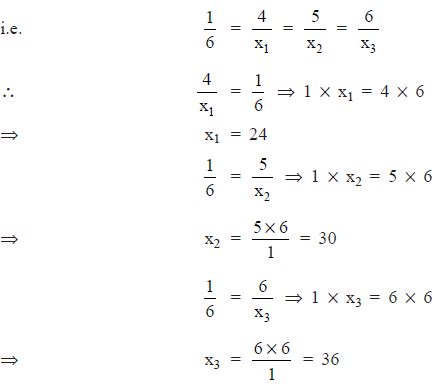Thus, the required unknown quantities are: x1 = 24, x2 = 30, and x3 = 36.

Question 2: A machine fills 540 bottles in six hours. How many bottles will it fill in five hours?
Solution:

 Numbers of bottles filled Number of hours 540 6 x 5

Let the required number of bottles to be filled in 5 hours = x
Since, more number of bottles, more number of hours would be required.
∴ The given quantities very directly.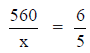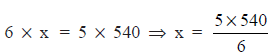= 5 * 90 = 450
Thus, the required number of bottles = 450.

Question 3: Jagmeet has a road map with a scale of 1 cm = 20 km. He drives on a road for 72 km. What would be his distance covered in the map?
Solution: Let the distance covered on the map be ‘x’ km.
∴ We have:

 Actual distance covered on the road (in km) Distance covered (represented) on the map ( in cm) 20 1 72 x

It is a case of direct variation.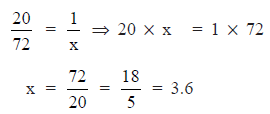Thus, the required distance on the map is represented as 3.4 cm.

Question 4: In a PG House, the food provision for 20 persons is for 10 days. How long would the food provision last if there were 5 more persons in that PG house?.
Solution: Number of persons added = 5
∴ Present number of persons = 20 + 5 = 25
Since, for more persons, the food will last less number of days.
∴ It is a case of inverse variation.
∴25 * x = 20 * 10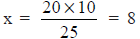∴ The food will now last for 8 days.

Question 5: A contractor estimates that 5 persons complete a task in 4 days. If he uses 4 persons instead of 5, how long should they take to complete the task?
Solution: More is the number of persons, less is the time to complete the task.
∴ It is a case of inverse variation,
Now, we have:

 Number of presons Number of days to complete the task 5 4 4 x

5 * 4 = 4 * x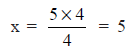Thus, the required number of days = 5.

Question 6: A school has 9 periods a day each of 50 minutes duration. How many period will there be, if the duration of every period is reduced by 5 minutes?
Solution: Presents duration each period = (50 – 5) minutes = 45 minutes.
Let the present number of period be ‘x’.
Since, more the number of periods, less is the duration of a period.
∴ It is a case of inverse variation.
We have: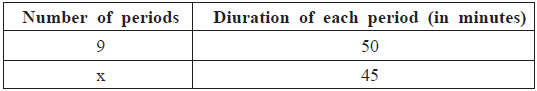∴  9 * 50 = x * 45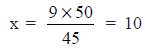Thus, the required number of periods = 10.

The document Important Questions: Direct and Inverse Proportions Notes | Study Mathematics (Maths) Class 8 - Class 8 is a part of the Class 8 Course Mathematics (Maths) Class 8.
All you need of Class 8 at this link: Class 8

## Mathematics (Maths) Class 8

187 videos|321 docs|48 tests
 Use Code STAYHOME200 and get INR 200 additional OFF

## Mathematics (Maths) Class 8

187 videos|321 docs|48 tests

Track your progress, build streaks, highlight & save important lessons and more!

,

,

,

,

,

,

,

,

,

,

,

,

,

,

,

,

,

,

,

,

,

;